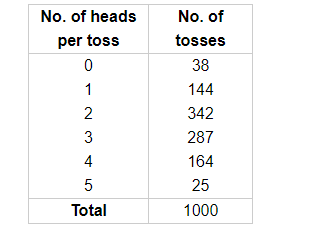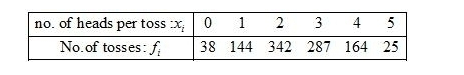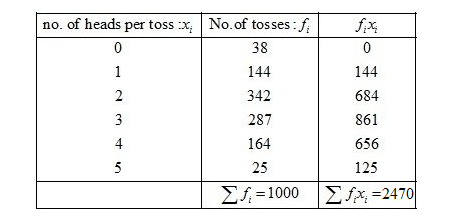# Five coins were simultaneously tossed 1000 times

Question:

Five coins were simultaneously tossed 1000 times and at each toss the number of heads were observed. The number of tosses during which 0, 1, 2, 3, 4 and 5 heads were obtained are shown in the table below. Find the mean number of heads per toss.Solution:

Given:First of all prepare the frequency table in such a way that its first column consist of the numnber of heads per tosses $\left(x_{i}\right)$ and the second column the corresponding number of tosses $\left(f_{i}\right)$.

Thereafter multiply the frequency of each row with corresponding values of variable to obtain third column containing $\left(f_{i} x_{i}\right)$.

Then, sum of all entries in the column second and denote by $\sum f_{i} x_{i}$ and in the third column to obtain $\sum f_{i} x_{i}$.We know that mean, $\bar{X}=\frac{\sum f_{i} x_{i}}{\sum f_{i}}$

$=\frac{2470}{1000}$

$=2.47$

Hence, the mean number of heads per toss is 2.47.